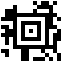Aztec Code is a type of 2D barcodes. It was initially published as US Patent 5591956 and is also covered in ISO/IEC 24778:2008.

### Generation

Aztec Codes can easily be generated from python by using the treepoem library as shown below.

``````import treepoem
import sys

if len(sys.argv) == 3:

print "Generating Aztec code"
print "Data: " + sys.argv
print "Filename: " + sys.argv
aztec = treepoem.generate_barcode(barcode_type='azteccode',data=sys.argv,o\$
aztec.save(sys.argv)

else:
print "Parameter Error!"
print "gen_aztec.py \"filename\" \"data\""
``````

### Decoding

The best solution for decoding Aztec Codes from python I was able to find is a ZXing wrapper called python-zxing.

``````from zxing import *
import sys

zx = BarCodeReader("/opt/tools/zxing/")

if len(sys.argv) == 2:

print "Opening file " + sys.argv + "\n\n"

barcode = zx.decode(sys.argv,True)

if barcode != None:

print "Printing raw\n"
print barcode.raw
print "\n\nPrinting data\n"
print barcode.data

data = open(sys.argv+".raw.txt",'w')
data.write(barcode.raw)
data.close()

data = open(sys.argv+".data.txt",'w')
data.write(barcode.data)
data.close()

else:
print "No code found"

else:
print "Argument error!"
print "python dec_aztec.py \"imagename\""

``````

### Examples

#### Small Code

Code containing the text `Aztec Test`#### Large Code

Code containing the begining of H.P Lovecraft’s The Shadow over Insmouth